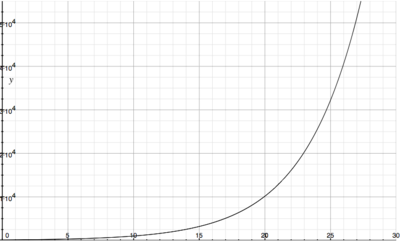# Under ideal conditions a certain bacteria population is known to double every three hours....

## Question:

Under ideal conditions a certain bacteria population is known to double every three hours. Suppose that there are initially 100 bacteria.

(a) What is the size of the population after 15 hours?

(b) What is the size of the population after {eq}t{/eq} hours?

(c) Estimate the size of the population after 20 hours.

(d) Graph the population function and estimate the time for the population to reach 50,000.

## Exponential Growth and Doubling Time

One type of model for exponential growth involves knowing the doubling time of a quantity. If we know both this doubling time, d, and the initial value of this quantity, {eq}P_0 {/eq}, we can define the following function calculating the value at any time.

{eq}P(t) = P_0 \cdot 2^{\frac{t}{d}} {/eq}

a) Since the population doubles every 3 hours, we can find the population after 15 hours by realizing that the population doubles 5 times in this first 15 hours:

t P
0 100
3 200
6 400
9 800
12 1600
15 3200

Thus, there are 3200 bacteria after 15 hours.

b) Let's construct the model that calculates this population at any time. We have the initial amount of bacteria in this culture, 100, and the doubling time, 3 hours, so we can find this model as follows.

{eq}P(t) = 100 \cdot 2^{\frac{t}{3}} {/eq}

c) We can use the model we defined in part b to find the population after 20 hours.

{eq}P(20) = 100 \cdot 2^{\frac{20}{3}} = 10159.3667 {/eq}

Thus, there are approximately 10,159 bacteria after 20 hours.

d) With this function defined, we can graph it. This can be used to approximate the time that it would take for the population to reach 50,000, by seeing when the function reaches this value.